## By Pavel Panchekha

### 21 June 2015

Share under CC-BY-SA.

# Zippers, Part 4: Multi-Zippers

###### Series

This is post 4 of the Zippers series.

Zippers are a way to update a data structure in a purely functional way. It replaces implicit motion across a data structure with an explicit cursor. This last of four parts describes a method to update a data structure quickly at several points.

## Multiple cursors

In previous parts, I described three views on zippers: the classic list and tree zippers described by Huet; zippers as data structure derivatives; and zippers as program derivatives using continuations. In all of these cases, the zipper in question allowed locally editing a data structure in $$O(1)$$ time, where by locally I mean within $$O(1)$$ distance of some fixed location.

In this part, I'll describe some results that I think are novel, generalizing the zipper to allow updates within $$O(1)$$ distance of one of $$k$$ fixed locations. The final runtime is $$O(k \log k)$$ per update—notably, this is independent of the size of the data structure, just like for simple one-hole zippers. This blog post is based on my term paper for MIT's 6.854 Advanced Algorithms class, but with errors corrected and the presentation improved. Nonetheless, this post is a little stuffier than my usual post; sorry!

Why would you want multiple zippers? Imagine an XML editor, where users are mostly making updates around a cursor location, and where we need to store multiple copies for "undo" functionality. Or imagine a versioned file system, where most changes are made near the current directory, and where multiple users might be working at once. I'm not talking about concurrency here1 [1 Though the initial goal of this work was automatic eventual consistency.]; all operations are totally ordered. But we want to make edits quickly, and we want to store old versions persistently.

To generalize the usual zipper framework, we'll associate each user with their own “cursor”, which they can move around the data structure. The data structure of interest is a tree with extra data at each node,2 [2 A tree of bounded degree; if the degree were unbounded, just moving along edges might take a while] so imagine that a cursor can move along any edge, or change the data at the node. I'll refer to some small region where multiple operations happen as a locality or region, which we assume to be $$O(1)$$ in diameter; think of this as a part of the data structure that a cursor “owns”.

## Multiple zippers, multiple regions

The goal is to generalize the above construction for updates that occur in multiple places. One approach, of course, would be to simply move one cursor back and forth between places; however, since the places may be as much as $$O(n)$$ apart, this quickly destroys any runtime guarantees we can make. So instead, I'll use a more complex data structure. Luckily, it doesn't end up being particularly complex to analyze. The construction does require solving many fun but reasonably simple problems. This construction is best read as successive approximations to a working data structure; the summary at the end may be helpful as an overview of the final, resulting, structure.

Imagine deleting some of the links in the tree, partitioning the tree into connected regions, such that every region contains one locality. If we preserve information about edges that used to exist, we can reconstruct the full tree from these regions. Each region is itself a tree, so you can use a standard zipper in each region, responsible for operations in that region.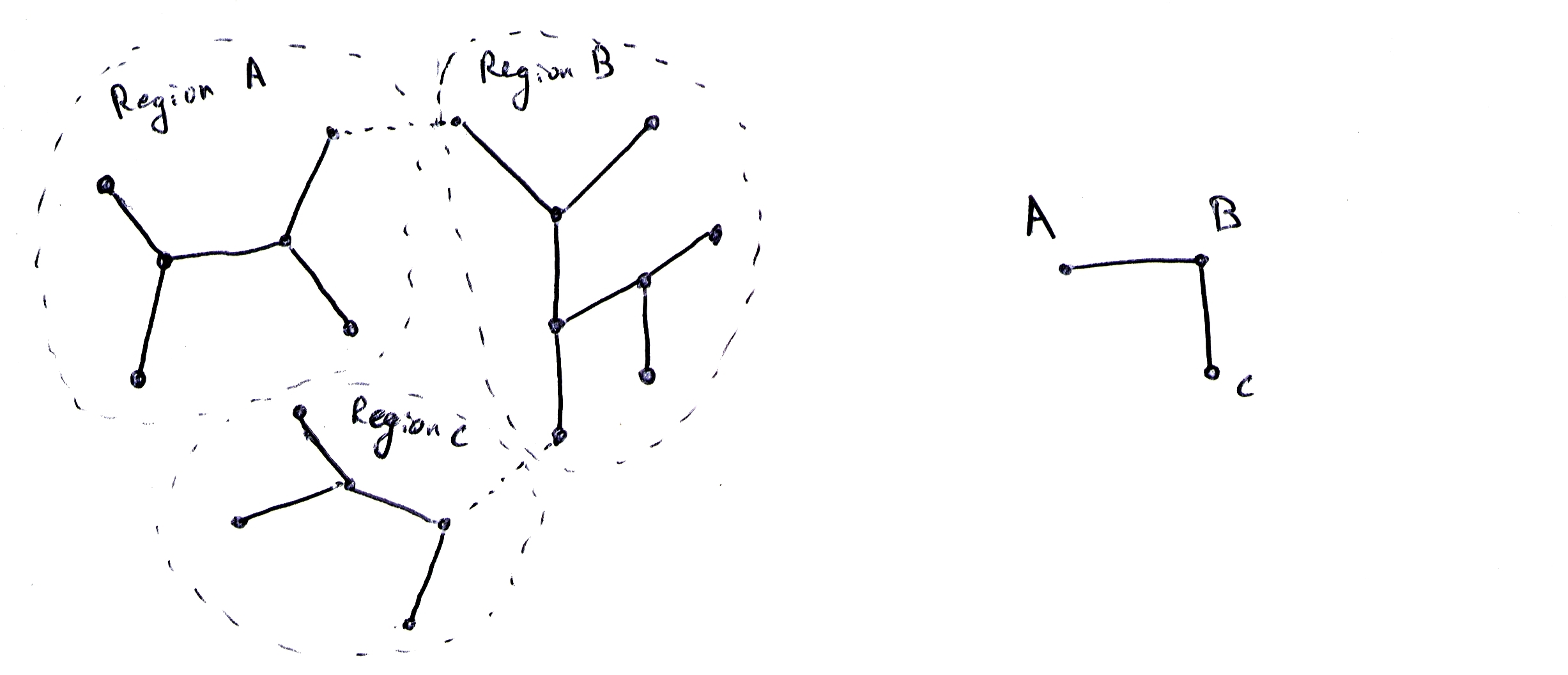Figure 1: Dividing a tree into regions, and the associated region tree.

This is the overall structure of the multi-zipper: partition the overall tree into regions, and store one zipper per region. This approach requires solving two problems: first, maintaining information about how regions are linked; and second, repartitioning the tree when cursors move around the tree.

## Repartitioning

First, let's solve the problem of efficiently repartitioning the tree as cursors move about. Suppose a cursor reaches the edge of its region and attempts to move beyond this border. To satisfy this request, we use the (undiscussed) data structure for linking regions to figure out what region the cursor is moving into, and find the path between the moving cursor, and the cursor in charge of the region we're moving into. We can then split this path in half. Since we're talking about a tree, where removing any edge splits the tree, splitting the path in half also splits the region in half. We can leave the half closer to the moving cursor with the moving cursor, and the half closer to the other cursor with it.

Now, the whole path used to point away from the other cursor and toward the moving cursor, so the half that is being given to the moving cursor will have to be modified to flip the direction of its edges. But we don't have to change any of the other edges in this half-region: they pointed away from the old cursor, so away from this path, which is still the correct direction to point. 2 is a picture showing this reverse step. The splitting and reversing can be done in $$O(\ell)$$ ($$\ell$$ being the length of the path) time by copying the relevant nodes. Luckily, this operation only needs to be done every $$O(\ell / 2)$$ operations, because it only happens when we reach the edge of a region; so this is $$O(1)$$ amortized time.3 [3 I'll make this worst-case in just a bit.]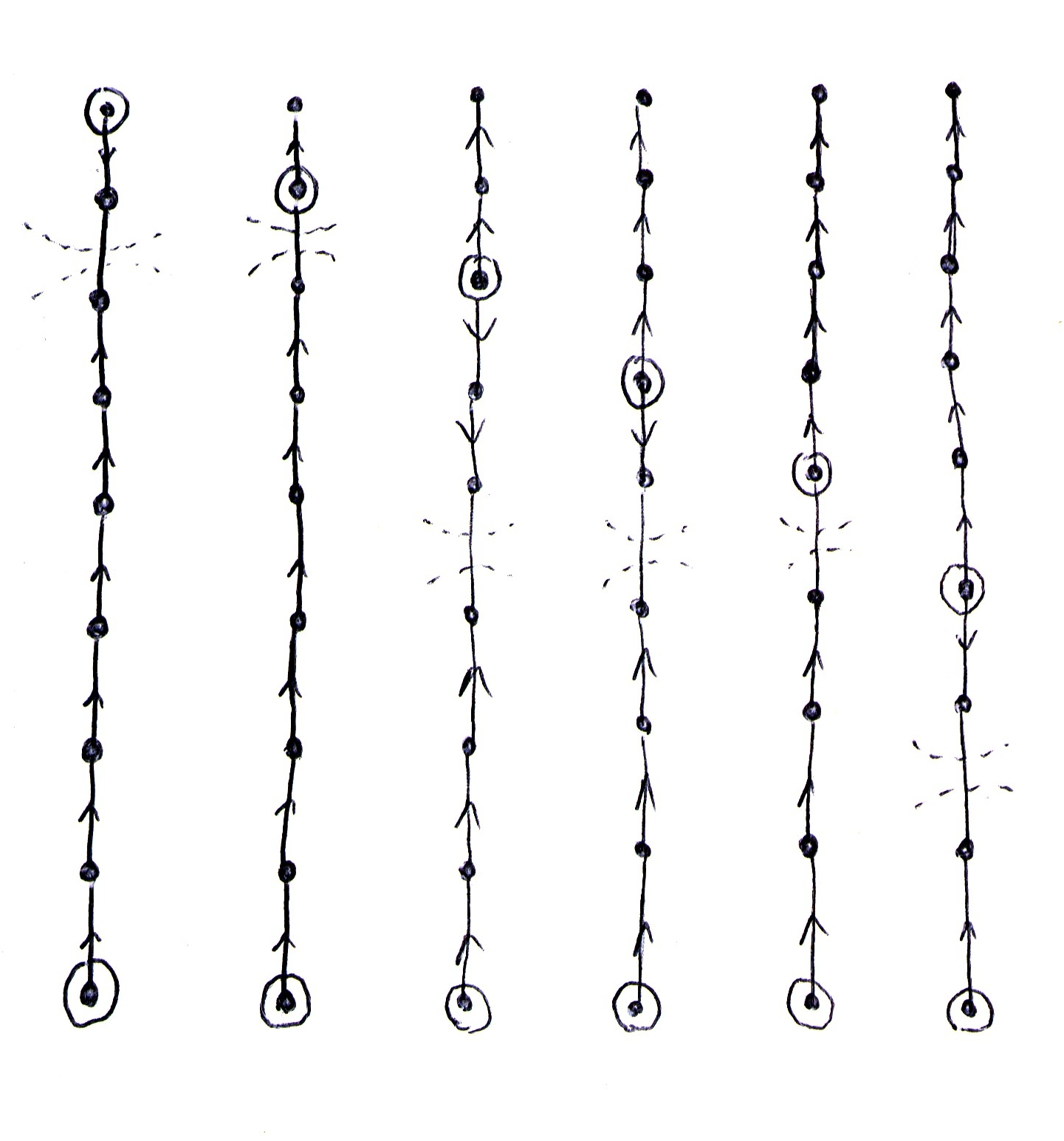Figure 2: Repartitioning regions as one cursors moves toward another.

To keep the calculation of the path fast, we keep the path from a cursor to each border of its region in a separate linked list; we can update this path in $$O(1)$$ time every time either cursor moves, since we are on a tree. That is, each node will explicitly store the path from a cursor to any of its neighboring regions, and update each such path as it moves.

What happens if one cursor moves onto the node pointed to by another cursor? I solve this problem by imagining that each cursor that we present to data structure users is really a handle; multiple handles at the same place may be backed by a single physical cursor, which stores a count of how many handles it backs. (I'll refer, from now on, to the physical cursors as "cursors" and the virtual handles as "handles".) This way, if one cursor modifies a location being pointed to by another cursor, you don't have to go and modify every cursor looking at this location to store a new value.

When one handle moves onto a node pointed to by another handle (when two cursors "merge"), you simply assign all handles of either cursor to point at the same cursor, and update that cursor's count. So I need to keep track of which handle maps to which cursors; I'll talk about that in a later section.

I'm only going to sketch the algorithm here; actually implementing it would be a decent amount of work, especially since the data structure is pretty complex, and since I'm not going to go into full detail on what each operation looks like.

I've discussed how to make updates within a region, but I also need to track adjacency information for regions. This information is necessary for rebuilding the original tree if ever necessary and for repartitioning efficiently.

So imagine taking the original tree and contracting each region to a point. This yields a tree again,4 [4 It is a tree, not a graph; this is crucial for the multi-zipper being fast.] representing the linkage of regions to one another. So I will track and update this region tree together with the actual tree, and use it for determining adjacency information between regions. Note that for a tree with $$k$$ cursors, the region tree or cursor tree has size $$O(k)$$.

Usually, repartitioning affects the adjacency information for only the two regions whose border is being moved. However, for certain edge cases this is not true. When a cursor moves into the point between multiple regions, there could be a lot of changes happening to the region tree, and I want to limit this change to make updating the region tree efficient.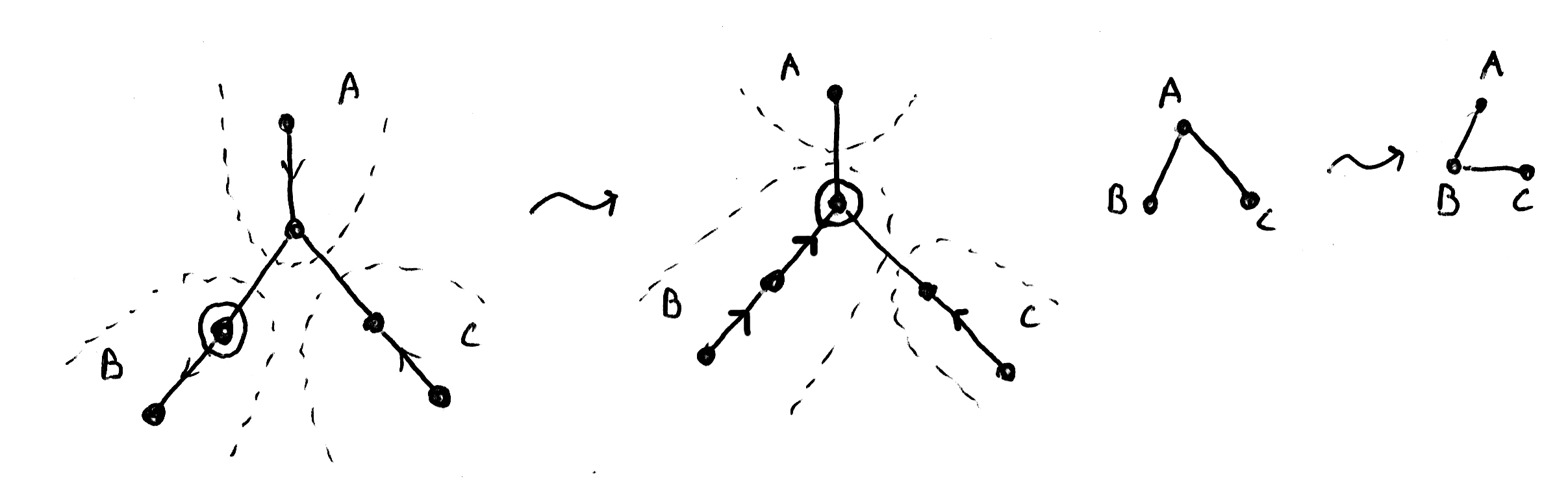Figure 3: A repartitioning affecting multiple regions, a problem we solve with ghost cursors (also, the associated region trees).

To limit the changes which can happen to the region tree, I'm going to insert extra cursors, with their own associated regions, so that all updates to the region tree involve only two regions. These "ghost cursors" have to occur at branch points—points at which there are cursors in three or more different directions.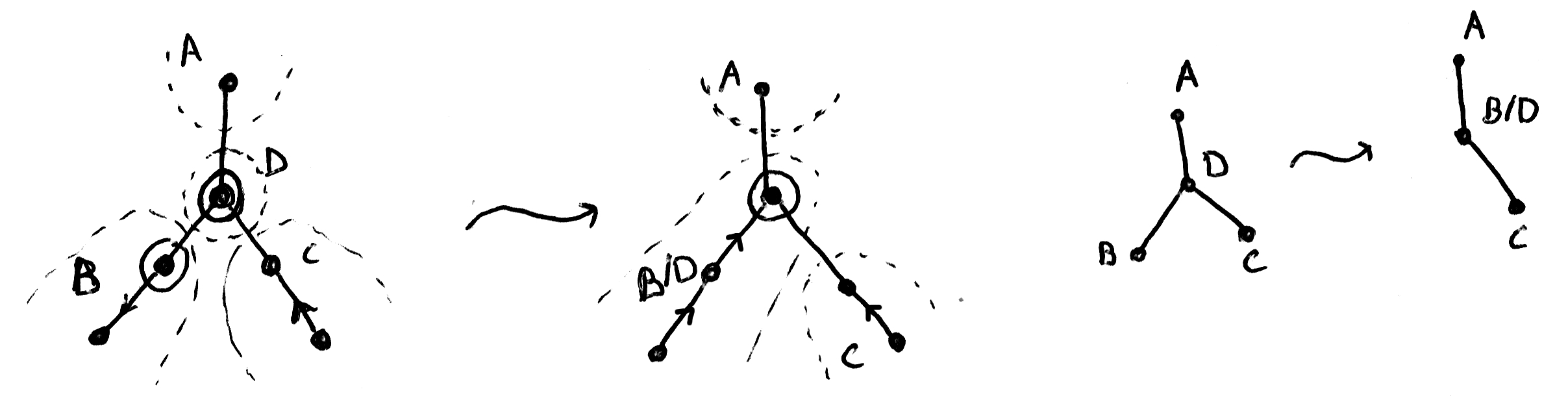Figure 4: The same structure but with the ghost cursor $$D$$; and the region trees of the same.

Thankfully, there are $$O(k)$$ such points (see Lemma 1), so adding these ghost cursors doesn't make the region tree too big. It's easy to represent ghost cursors in the region tree: they are cursors that back zero handles. Here are some cases where ghost cursors are necessary: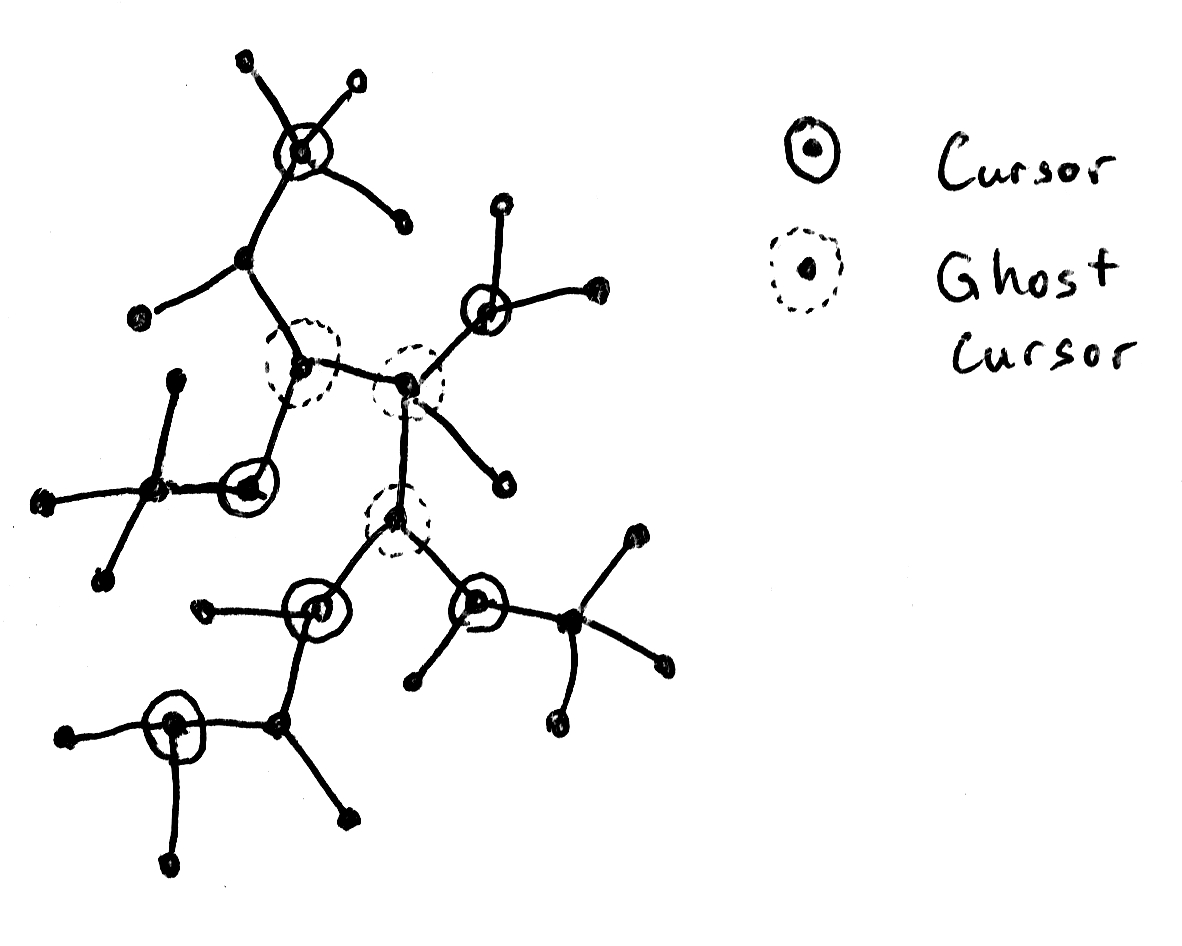Figure 5: Cursors and ghost cursors.

Lemma 1: Only $$O(k)$$ ghost cursors are needed to mark all branch points in a tree of $$k$$ cursors. More formally, given $$k$$ marked cursors in a tree, one can mark $$O(k)$$ extra nodes, so that the paths between adjacent marked nodes (two marked notes where the path between them does not pass through a marked node) are disjoint.

Proof: By induct on the number of marked nodes. Choose two marked leaves with least common ancestor furthest from the root. Add this least common ancestor as a marked ghost node. Any paths from any marked node to either chosen node must pass through this ghost node, so the chosen node are adjacent only to this newly marked node; but the paths between the newly marked node and the chosen nodes are disjoint by construction. So ignore the two chosen nodes. We now have $$k - 1$$ marked nodes total ($$k-2$$ originally-marked nodes, and one additional ghost node). Induct. In the base case, with one cursor, I need zero ghost nodes; so with $$k$$ cursors we need at most $$k - 1$$ ghost nodes. \box

Now, when must one update the region tree? Just repartitioning regions does not affect the region tree, since the relationship between the two cursors is still the same. But when two cursors merge (see, again, Figure 4), or when a new ghost cursor has to be added the region tree changes. The region tree can be updated in $$O(k)$$ time by copying.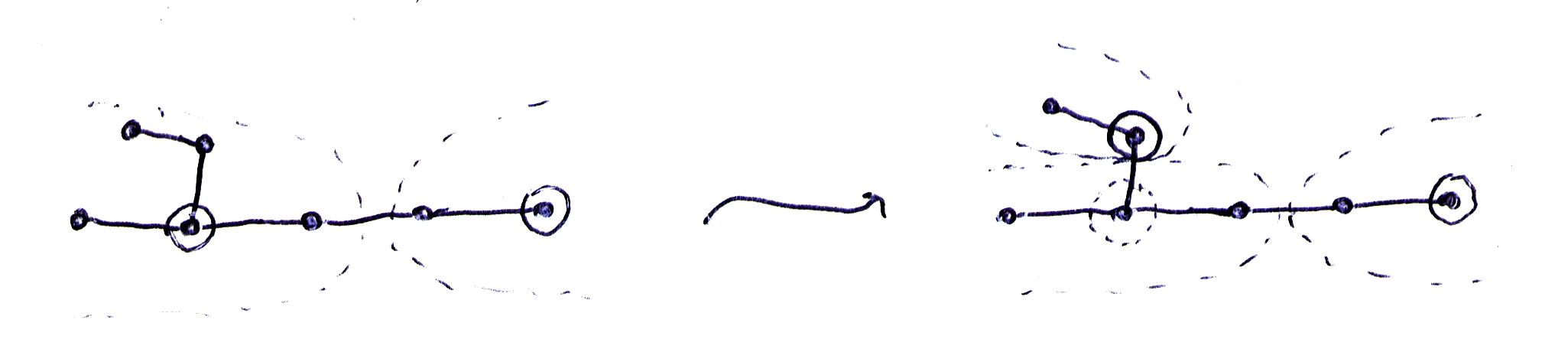Figure 6: Adding a ghost cursor due to normal cursor motion.

## Mapping Virtual to Actual Cursors

So now we've got a $$O(k)$$ cursors, arranged in a tree, and each backing zero or more handles, and each controlling a region of the tree. Moving a handle might require: repartitioning a region; splitting or merging cursors, requiring edits to the region tree; or moving a cursor in a way that requires adding a ghost cursor.

To keep track of which handles are backed by which cursor, we need a way to map handles to cursors in a persistent way. If a cursor backs multiple handles, and that handle makes a change, it has to persistently update the cursor, and thus update what cursor each handle points to.

You can do this by storing a persistent map of some sort (likely a balanced tree from Okasaki's Purely Functional Data Structures) from handles to actual cursors. This map takes $$O(\log k)$$ time per update, and we may have to change $$O(k)$$ entries (if, for example, all $$k$$ handle are backed by one cursor); so our runtime for each operation may be $$O(k \log k)$$.

To know which cursors to update, you either need to store a list of handles per cursor, or just go through the whole tree, updating if necessary. Either way works, and has the same asymptotics, so the choice would depend on practical considerations.

## Removing Amortization

So far I've described an amortized $$O(k \log k)$$ running time. The amortization is there because of repartitioning the regions when a cursor moves to the boundary of a region. However, this amortization cost can be removed. After all, this splitting and reversing behavior is really just an instance of real-time persistent dequeues. You can implement such a thing using scheduling, as described in Okasaki's book. You can use the recursive-slowdown queues to remove the amortization: store the paths between adjacent cursors in recursive-slowdown queues, and do away with an explicit repartitioning step. This makes our runtime a worst-case $$O(k \log k)$$, finishing the multi-cursor zipper construction.5 [5 With this change, the notion of explicit regions is obsolete. Instead, imagine the tree nodes between two cursors as graded from being "owned" by one cursor to being owned by the other.]

Where are these queues stored? They can be stored at the cursor at either end, in which case any operation to a queue will have to update the cursors at each end to point to the new queue; or, it can be held in a new map, with cursors pointing into it. The second is obviously more efficient, but more complex, so I'm going to assume the first variant here.

## Data Structure Summary

The design of multi-zippers is rather complex, I'll restate the overall data structure.

An order $$k$$ multi-zipper of a tree is simply a collection of $$O(k)$$ cursors and a map from $$k$$ handles to the cursors. Each cursor consists of some number of recursive-slowdown queues, labeled by a direction, where each queue represents a path from the cursor to the adjacent cursor in that direction; this path consists of nodes plus nodes attached to those nodes, called dangling subtrees. Parts of the tree might not be on the way between two cursor; these are called attached subtrees and are stored at the cursor they are closest to. Finally, each cursor stores the node the cursor is atop of. Each cursor is also stores the count of handles pointing to it. This count may be zero, since some cursors are "ghost cursors". The collection of cursors is stored in a region tree—the tree formed by contracting all regions into single points.

To use the data structure, one sends commands to handles which either move it or manipulate the node it is atop. To manipulate the node, the handle is dereferenced to get a cursor, and the cursor's current node is changed.

Moving the handle is more complex. First, it is dereferenced to get its backing cursor. Now one of many cases can occur:

### If the cursor has count 1

#### If the cursor is adjancent to 1 cursor, and the motion is toward that cursor, and the queue between them is nonempty

One node is popped from that queue. It becomes the current node, and its dangling subtrees become the new attached subtrees. The old current node is joined with all attached subtrees and becomes another attached subtree.

#### The cursor is adjacent to 1 cursor, and the motion is toward that cursor, and the queue between them is empty

The current node is joined to all attached children and becomes an attached child of the adjacent cursor. The handle is updated to point to the adjacent cursor, whose count is increased.

#### The cursor is adjacent to 1 cursor, and the motion is not toward that cursor

Add the current node to the queue, with the current attached trees, except the one being moved into, as dangling trees. Take the attached tree being moved into; make its root the current node and its subtrees the attached subtrees.

#### The cursor is adjacent to 2 cursors, and the motion is toward one

Put the current node plus attached subtrees into the queue being moved toward; pope a node from the queue being moved away from, and make its dangling subtrees the new attached subtrees.

#### The cursor is adjacent to 2 cursors, and the motion is away from both

Decrement the cursor's count (to 0) but leave it in place. Remove the attached subtree being moved into; make its root a new cursor (of count 1) with its subtrees as attached trees. Both the new and old cursor should gain an additional queue (between them) with no nodes in it. The handler used should now point to the new cursor.

### If the cursor has count > 1

#### The motion is toward some other cursor

Pop a node off that queue, make it the root of a new cursor (with the dangling subtrees the new attached subtrees), which has two queues: the one just popped, and a new empty queue. The other end of that queue is held by the old cursor instead of the queue it used to have. Update the handle to point to the new cursor.

#### The motion is not toward some other cursor

Add a new cursor, whose root is the attached subtree being descended into, and whose attached subtrees are the subtrees of this root; add a queue between the new and old cursor, and remove the attached subtree from the old cursor. Update the handle to point to the new cursor.

## Conclusion

This mess of cases does appear dreadfully complex, but I think a few minutes playing with each one on paper will make things clear. Each operation involves at most manipulating 3 cursors, updating $$O(k)$$ handles, and doing 2 queue operations, for a maximum runtime of $$O(k \log k)$$.6 [6 Besides manipulating nodes and moving handles, operations for cloning and deleting handles could be added by just manipulating the handle to cursor mapping.] So this data structure allows updating a tree in multiple locations, fully persistently, in time independent of the size of the tree.

## Footnotes:

1

Though the initial goal of this work was automatic eventual consistency.

2

A tree of bounded degree; if the degree were unbounded, just moving along edges might take a while

3

I'll make this worst-case in just a bit.

4

It is a tree, not a graph; this is crucial for the multi-zipper being fast.

5

With this change, the notion of explicit regions is obsolete. Instead, imagine the tree nodes between two cursors as graded from being "owned" by one cursor to being owned by the other.

6

Besides manipulating nodes and moving handles, operations for cloning and deleting handles could be added by just manipulating the handle to cursor mapping.